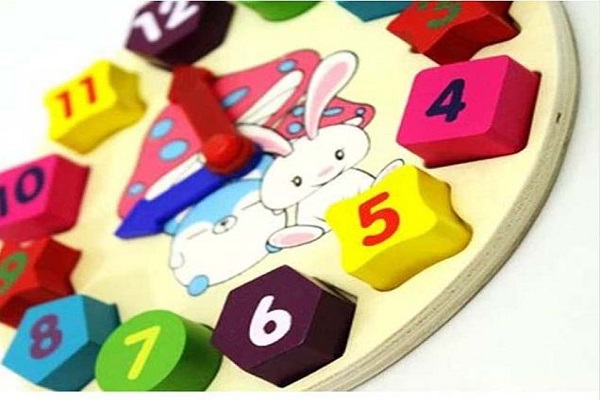# How to learn dozens tips and exercises

Here we will show that some forms to explain the tens to the students. They must already know or have an idea of the notion of “unity” to continue. You will find that for some children, dozens are more difficult to assimilate than you think, be patient and follow the steps below to learn how to teach dozens.

##Count and form groups of 10

Prepare on the computer or with paper and pencils, different graphics, such as those shown below. Students must count 10 points and surround them with a pencil mark. How many groups of 10 points are on the drawing? How many units remain?

It is recommended that the number of groups that can be formed does not exceed 10. Students should write the numbers in the appropriate boxes.

You may also like to read: University in England: The British Council gives you a hand

###This is the moment to explain to the student:

• 1 group of ten = 1 dozen = ten
• 2 groups of ten = 2 tens = twenty
• 3 groups of ten = 3 tens = thirty
• 4 groups of ten = 4 dozen = forty
• 5 groups of ten = 5 tens = fifty
• 6 groups of ten = 6 tens = sixty
• 7 groups of ten = 7 tens = seventy
• 8 groups of ten = 8 tens = eighty
• 9 groups of ten = 9 tens = ninety
• 10 groups of ten = ten dozen = one hundred

##The counting game

You will need matches or toothpicks initially place 10 matches on the table. In the game, each player adds a match to the common stock on the table, and says the number of the number. Each time the player puts a match that forms a set of ten years; the ten matches are assembled with an elastic.

In the first version, the rule is that you can only use words from one to ten, if included. In other words, do not use numbers like eleven, thirteen, twenty, etc. For example: a player puts match number 11 and instead of saying eleven , he says “a dozen and one”, twelve becomes “ten and two”, twenty then corresponds to “two dozen”.

Then you can change the game so that, in turn, each player adds to the stack two matches instead of one. Alternatively, that each one adds even three. It is a good way to count!

Then start the game again, but this time, players will have to tell the number of the two ways, the usual and the detailed.

Finally, start with a large stack of matches, about 100. Each player must take two or three matches and tell the remaining number.

If you would like to read more articles like education , we recommend our education category.